Next: Bibliography Up: 3. Reference Manual Previous: 20. The Initial Theory

# Glossary

#### 20.0.0.0.1 Alpha-equivalence

Two expressions are alpha-equivalent if one can be obtained from the other by renaming bound variables.

#### 20.0.0.0.2 Application

An expression whose lead constructor is named apply. Applications must have at least two components. The first component of an application is called the operator and the remaining components are called arguments. Applications are usually denoted by prefixing the operator to the arguments enclosed in parentheses as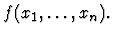However, other forms (such as infix for algebraic operations) are also used.

#### 20.0.0.0.3 Beta-reduction

An inference rule which plugs in the values of lambda-applications. For example, beta-reduction transforms the expression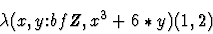to 13.

#### 20.0.0.0.4 Buffer

An Emacs data structure for representing portions of text, for example, text files which are being edited. To the user, a buffer has the appearance of a scrollable page. More than one buffer may exist at a given time in an Emacs session. Users will also use buffers to build, edit and evaluate def-forms.

#### 20.0.0.0.5 Command form

An s-expression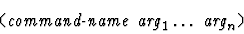used in proof scripts. The number and type of arguments is command-dependent. If the command takes no arguments, then the form command-name is also valid.

#### 20.0.0.0.6 Compound expression

An expression with a constructor and a (possibly empty) list of components. 1+2is a compound expression whose constructor is named apply and whose components are +,1,2.

#### 20.0.0.0.7 Constant

A kind of IMPS expression. For example, in the theory named h-o-real-arithmetic, the expressions 1,2,+ are constants.

#### 20.0.0.0.8 Constructor

One of the 19 logical constants of LUTINS. Constructors are used to build compound expressions.

#### 20.0.0.0.9 Context

An IMPS data structure representing a set of of formulas to be used as assumptions. Contexts contain various kinds of cached information (for example, about definedness of terms), used by the IMPS simplification machinery.

#### 20.0.0.0.10 Core

All the basic logical and deductive machinery of IMPS on which the soundness of the system depends.

#### 20.0.0.0.11 Deduction graph

An IMPS data structure representing a proof. Deduction graphs contain two kinds of nodes: inference nodes and sequent nodes.

#### 20.0.0.0.12 Def-form

An s-expression whose evaluation has some effect on the IMPS environment, such as building a theory, adding a theorem to a theory, making a definition in a theory or building a translation between theories. There are about 30 such def-forms.

#### 20.0.0.0.13 Emacs

A text editor. Although various implementations of Emacs exist, in this manual we mean exclusively GNU Emacs, which is the extensible display editor developed by the Free Software Foundation. Because of the many extension facilities it provides (including a programming language with many string-processing functions), GNU Emacs is well-suited for developing interfaces.

#### 20.0.0.0.14 Expression

An IMPS data structure representing an element of a language. Expressions are used to describe mathematical entities or to make logical assertions. Two examples (printed in TEX) are 22n-1 and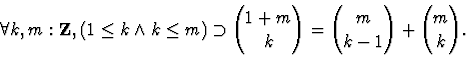An expression is either a formal symbol or a compound expression.

#### 20.0.0.0.15 Evaluate

To cause an s-expression to be read by the T process, thereby creating an internal representation of a program, which is then executed by T. This sequence of events usually has a number of side-effects on the IMPS environment. For example, to define a constant named square in the theory h-o-real-arithmetic, evaluate the s-expression:

(def-constant SQUARE
"lambda(x:rr, x^2)"
(theory h-o-real-arithmetic))


#### 20.0.0.0.16 Formal symbol

A primitive IMPS expression. A formal symbol has no components, although it is possible for an expression with no components to be a compound expression. A formal symbol can be a variable or a constant. Every formal symbol has a name which is used for display. The name is a Lisp object such as a symbol or a number.

#### 20.0.0.0.17 Formula

Intuitively, an expression having a truth value, for example,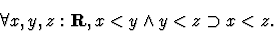In IMPS a formula is an expression whose sort is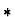.

#### 20.0.0.0.18 IMPS

A formal reasoning system and theorem prover developed at The MITRE Corporation.

#### 20.0.0.0.19 Inference node

A node in a deduction graph, connecting a conclusion sequent node with zero or more hypothesis sequent nodes, that represents an instance of a rule of inference.

#### 20.0.0.0.20 Iota-expression

A compound expression whose lead constructor is named iota, denoted in the mathematical syntax by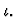An iota-expression has the form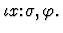It denotes the unique object in the sort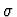which satisfies the condition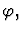if such an object exists. Otherwise, the iota-expression is undefined.

#### 20.0.0.0.21 Kind

Each sort and expression is of kind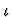(also written as iota) or(prop). Expressions of kindare used to refer to mathematical objects; they may be undefined. Expressions of kindare primarily used in making assertions about mathematical objects; they are always defined.

#### 20.0.0.0.22 Lambda-application

An application whose operator is a lambda-expression. For example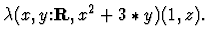#### 20.0.0.0.23 Lambda-expression

A compound expression whose lead constructor is named lambda, denoted in the mathematical syntax by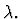A lambda-expression has the form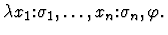It denotes the function of n arguments whose value at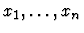is given by the value of termprovided the xi are of sort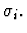#### 20.0.0.0.24 Language

Mathematically, a language in IMPS consists of expressions, sorts, and user-supplied information about sort inclusions. At the implementation level, a language is a Lisp data structure having additional structure which procedurally encodes this information. This is meant to facilitate various kinds of lower level reasoning, such as reasoning about membership of an expression in a sort.

#### 20.0.0.0.25 Little theories

A version of the axiomatic method in which mathematical reasoning is distributed over a network of theories linked to one another by theory interpretations, in contrast to the big theory'' approach in which all reasoning is performed within a single, usually very expressive theory.

#### 20.0.0.0.26 Local context

The set of assumptions relevant to a particular location in an expression.

#### 20.0.0.0.27 LUTINS

A version of type theory with partial functions and subtypes; the logic of IMPS.

#### 20.0.0.0.28 Macete

A user-defined extension of the simplifier. When a theorem is installed, it is also automatically installed as a macete. The way the corresponding macete works depends on the syntactic form of the theorem. In the simplest case in which the theorem is a universally quantified equality, the theorem is used as a rewrite rule. Macetes can be composed using the def-form named def-compound-macete. The word macete'' comes from Brazilian Portuguese, where it means clever trick.'' Theorem macetes can also be made into transportable macetes.

#### 20.0.0.0.29 Mode Line

The line at the bottom of each buffer window. It provides information about the displayed buffer such as its name and mode.

#### 20.0.0.0.30 Parser

A program which takes user input, usually represented as text, and builds a data structure suitable for computer processing.

#### 20.0.0.0.31 Primitive inference procedure

An IMPS procedure that implements one of the primitive rules of inference of the IMPS proof system. IMPS has about 30 primitive inference procedures.

#### 20.0.0.0.32 Proof

Conclusive mathematical evidence of the validity of some assertion. The rules for admissible evidence are given by a proof system.

#### 20.0.0.0.33 Quasi-constructor

A user-defined macro/abbreviation for building expressions. Quasi-constructors are used much like constructors.

#### 20.0.0.0.34 Quasi-equality

A quasi-constructor, written as infix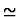in the mathematical syntax.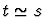means that either t and s are both undefined or they are equal.

#### 20.0.0.0.35 S-expression

The external representation of the basic data structure of Lisp-like languages including T. S-expressions are usually represented as nested lists such as (fuba (abacaxi farofa) alface).

#### 20.0.0.0.36 Sequent node

A node in a deduction graph that represents a sequent, a formula composed of a set of assumptions and an assertion.

#### 20.0.0.0.37 Sort

A sort denotes a nonempty set of mathematical entities. Every expression has a sort which restricts the value of that expression.

#### 20.0.0.0.38 Syntax

Rules for parsing and printing expressions. The syntaxes that are currently used in IMPS are the string syntax which allows for infix and postfix notation, the s-expression syntax which provides a uniform prefix syntax for all constructors and operators, and the tex syntax for displaying (but not parsing) in TEX.

#### 20.0.0.0.39 T

A dialect of Lisp developed at the Yale University Computer Science Department in which the core machinery of IMPS is implemented. T is very similar to the Scheme programming language, familiar to readers of Abelson and Sussman's book .

#### 20.0.0.0.40 Tea

An alias for the T programming language.

#### 20.0.0.0.41 Theory

The term theory'' as used in this manual, consists of a language and a set of closed formulas in the language called axioms. A theory is implemented as a record structure having slots for the language, the list of axioms, and other relevant information. There is also a slot for a table of procedures which the simplifier uses to apply the axioms. This is meant to facilitate various kinds of lower-level reasoning, such as algebraic or order simplification. In a different sense, theory is also commonly used to refer to a collection of related facts about a given mathematical structure, such as the theory of rings or the theory of ordinary differential equations.

#### 20.0.0.0.42 Theory ensemble

A data structure which bundles together theories which are unions of copies of a single base theory. Theory ensembles are mainly used to treat multiple instances of structures, such as triples of metric spaces or pairs of vector spaces.

#### 20.0.0.0.43 Theory interpretation

A translation that maps theorems to theorems.

#### 20.0.0.0.44 Translation

An IMPS data structure, created with the def-form named def-translation, that is used to translate expressions from one theory to another.

#### 20.0.0.0.45 Transportable macete

A theorem macete that can be used in a theory different from the one in which it was installed. Transportable macetes are named by the system by prefixing tr% to the name of the theorem.

#### 20.0.0.0.46 Type

A sort that is maximal with respect to the subsort relation.

#### 20.0.0.0.47 Variable

A kind of IMPS expression. An occurrence of a variable v within an expression is bound if it occurs with the body of a binding constructor which includes v as a binding variable. Otherwise, the occurrence of v is free.

#### 20.0.0.0.48 Xview

An IMPS procedure for printing expressions in TEX and then displaying them in an X window TEX previewer.Next: Bibliography Up: 3. Reference Manual Previous: 20. The Initial Theory